## 让 Draw2D 的组件也 Tween 起来

IBMdW 发布于 2011/06/20 20:51

Draw2D 作为 Java 2D 技术，用于绘制 Java 图形程序，目前主要是结合 GEF 在图形建模领域得到广泛应用，如 IBM 的 WBM（Websphere Business Modeler）。然而当我们熟悉 Draw2D 时，有时就会突然发觉 Draw2D 组件的单调，至少在笔者接触的很多 Draw2D 应用中，都没有使用到动画效果，与 Flex 开发的流程建模工具的对比，也促使读者研究了 Flex 的 Tween 技术，本文将 Flex 中的 Tween 概念以及相关算法引入到 Draw2D 当中，使得 Draw2D 具有一定的缓动效果（具体可以查看示例程序），读者可以将这些效果的算法应用到实际的 Draw2D 项目当中，相信可以使你的程序丰富多彩，吸引眼球。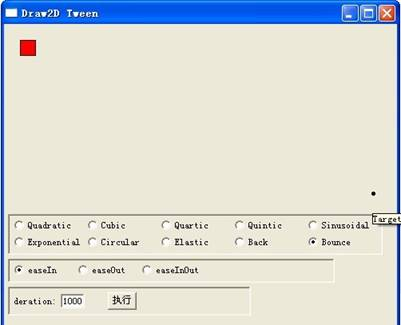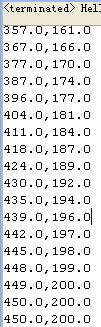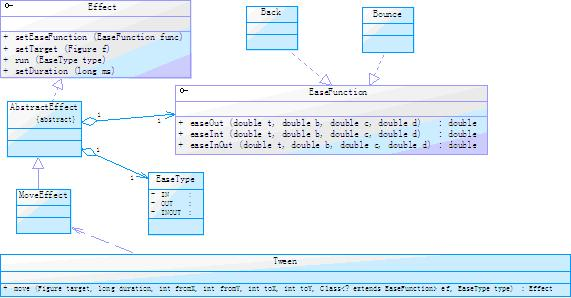```
public Effect move(Figure target, long duration, int fromX, int fromY, int toX, int toY,
Class<? extends EaseFunction> ef, EaseType type) {
MoveEffect e = new MoveEffect();
e.setFromX(fromX);
e.setFromY(fromY);
e.setToX(toX);
e.setToY(toY);
e.setTarget(target);
e.setDuration(duration);
try {
e.setEaseFunction((EaseFunction) ef.newInstance());
} catch (Exception e1) {
e1.printStackTrace();
}
e.run(type);
return e;
} ```

• double t：当前时间
• double b：初始值
• double c：变化量
• double d：持续时间

```
public class Bounce implements EaseFunction {

@Override
public double easeIn(double t, double b, double c, double d) {
return c - easeOut(d - t, 0, c, d) + b;
}

@Override
public double easeInOut(double t, double b, double c, double d) {

if (t < d / 2)
return easeIn(t * 2, 0, c, d) * .5 + b;
else
return easeOut(t * 2 - d, 0, c, d) * .5 + c * .5 + b;
}

@Override
public double easeOut(double t, double b, double c, double d) {
if ((t /= d) < (1 / 2.75)) {
return c * (7.5625 * t * t) + b;
} else if (t < (2 / 2.75)) {
return c * (7.5625 * (t -= (1.5 / 2.75)) * t + .75) + b;
} else if (t < (2.5 / 2.75)) {
return c * (7.5625 * (t -= (2.25 / 2.75)) * t + .9375) + b;
} else {
return c * (7.5625 * (t -= (2.625 / 2.75)) * t + .984375) + b;
}
}
} ```

MoveEffect 的 run 方法

```
private int fromX, fromY, toX, toY;

// 以下 3 个变量是在父类 AbstractEffect 中定义的

protected EaseFunction ease;// 用户选择的 easeFunction

protected Figure target;//MoveEffect 作用的 Figure 对象

protected long duration;// 用户输入的持续时间

@Override
public void run(final EaseType type) {
final Timer timer = new Timer();
long b = fromX, c = toX - fromX, d = duration / 10, t = 0;
long b2 = fromY, c2 = toY - fromY;
// 每隔 10 毫秒执行 MyTimer 的 run 方法
timer.schedule(new MyTimer(c, type, d, t, b, c2, b2), 0, 10);
}
private final class MyTimer extends TimerTask {
private long c;
private EaseType type;
private long d;
private long t;
private long b;
private long c2;
private long b2;
double curX = -1;
double curY = -1;

private MyTimer(long c, EaseType type, long d, long t, long b, long c2,
long b2) {
this.c = c;
this.type = type;
this.d = d;
this.t = t;
this.b = b;
this.c2 = c2;
this.b2 = b2;
}

@Override
public void run() {
// 判断用户选择的 Ease 类型
if (type == EaseType.IN) {

curX = Math.ceil(ease.easeIn(t, b, c, d));// 计算当前 X 坐标
curY = Math.ceil(ease.easeIn(t, b2, c2, d)); // 计算当前 Y 坐标
} else if (type == EaseType.OUT) {

curX = Math.ceil(ease.easeOut(t, b, c, d));
curY = Math.ceil(ease.easeOut(t, b2, c2, d));
} else if (type == EaseType.INOUT) {

curX = Math.ceil(ease.easeInOut(t, b, c, d));
curY = Math.ceil(ease.easeInOut(t, b2, c2, d));
}
if (t < d)
t += 1;// 刷新一次 t 要加 1
else
this.cancel();// 代表效果执行时间到，停止
if (target != null) {
Display.getDefault().asyncExec(new Runnable() {

@Override
public void run() {
// 刷新 target 的位置
target.setLocation(new Point(curX, curY));
}
});
}
// 打印到控制台
System.out.println(curX + "," + curY);
}
} ```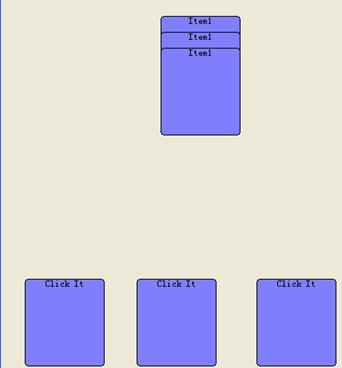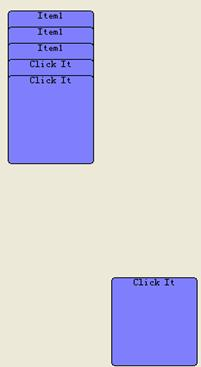```
final Figure cardItem = new RoundedRectangle();
cardItem.setLocation(new Point(320, 350));
cardItem.setSize(100, 110);
cardItem.setBackgroundColor(ColorConstants.lightBlue);
cardItem.setLayoutManager(new ToolbarLayout());
final Figure label = new org.eclipse.draw2d.Label("Click It");
cardItem.add(label);
cardItem.addMouseListener(new MouseListener() {
public void mousePressed(MouseEvent arg0) {
//lastPut 代表最近被移动到牌堆的那张牌
// 最关键：调用 Tween 的 move 方法，执行 move 效果
Tween.instance().move(cardItem, 1000,
cardItem.getLocation().x, cardItem.getLocation().y,
lastPut.getLocation().x,
lastPut.getLocation().y + 20, Bounce.class,
EaseType.IN);
lastPut = cardItem;
}
public void mouseReleased(MouseEvent arg0) {
}
public void mouseDoubleClicked(MouseEvent arg0) {
}
}); ```